# SSC CGL 2013 Tier 1 21 April Shift 2

Instructions

For the following questions answer them individually

Question 191

The above diagram shows if survey on a sample of 10, persons with reference their knowledge of English Hindi and Marathi. How man_ knew only Hindi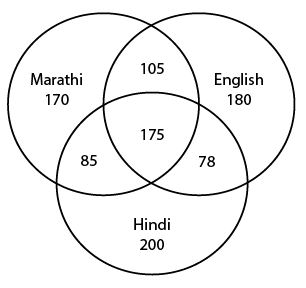Question 192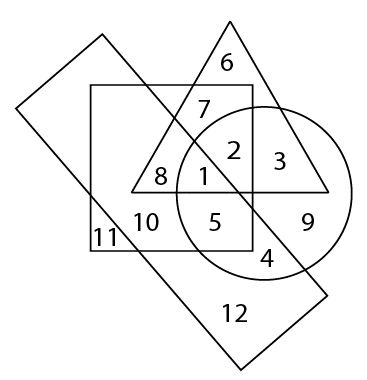In the above figure, the circle stands for employed, the square stands for social worker, the triangle stands for illiterate and the rectangle stands for truthful.Study the figure and answer the questions which region represents literate, employed people who are neither truthful nor social worker.

Question 193

Identify the answer figures from which the pieces given in question figure have been cut.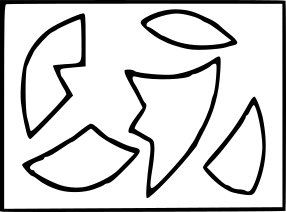Instructions

In the following questions, which answer figure will
complete the pattern in the question figure ?

Question 194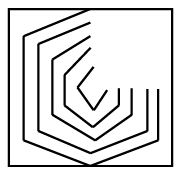Question 195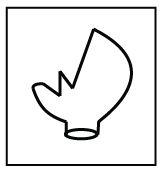Instructions

For the following questions answer them individually

Question 196

From the given answer figures, select the one in which the question figure is hidden/embedded.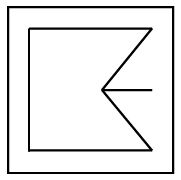Question 197

A piece of paper is folded and cut as shown below in the question figures. From the given answer figures, indicate how it will appear when opened.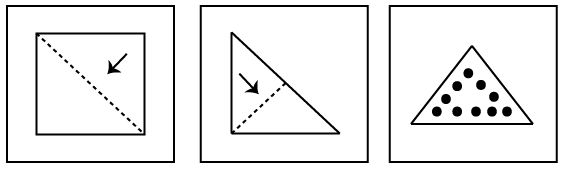Question 198

From the answer figures, find out the figure which is the exact mirror image of the question figure, when the mirror is place on the line ‘MN’ ?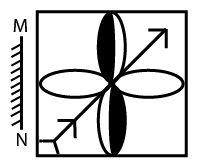Question 199

How many triangles are there in the given figure ?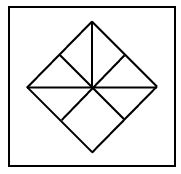Question 200

A word is represented by only one set of numbers as given in any one of the alternatives. The sets of numbers given in the alternatives are represented by two classes of alphabets as in two matrices given below. The columns and rows of Matrix I are numbered from 0 to 4 and that of Matrix II are numbered from 5 to 9 A letter from these matrices can be represented first by its row and next by its column, e.g., ‘A’ can be represented by 00, 12 etc. and ‘V can be represented by 56, 76 etc. Similarly, you have to identify the set for the word ‘PARROT.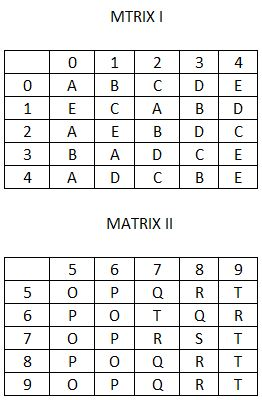OR